On anelliptic approximations for qP velocities in TI and orthorhombic mediaNext: Moveout approximation Up: Orthorhombic media Previous: Extended Muir-Dellinger Approximations

## Proposed Approximations

In the preceding section, we suggested an improvement to the anelliptic approximations for VTI media previously proposed by Fomel (2004). Applying a similar modification to the extended Muir and Dellinger approximations (equations 41 and 43), we can write the resultant approximations in a new form suitable for approximation of velocities in orthorhombic media, as follows:(45)

where(46)

and(47)

where(48)(49)(50)

Figure 3b shows the locations of the fitting indices in each symmetry plane. Note that the subscript rule explained earlier still applies, and the relationship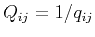still holds. In our notation,andrepresent weighted averages of anelliptic parameters (and) in a plane whereas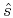andrepresent weighted averages at an axis.coordinate,fittingnotation
Figure 3.
a) Parametization rule for working coordinates. b) Locations of fitting indicesin each symmetry plane.Similar to the derivation in the TI case,andcan be found by fitting the curvatures to the exact velocities along orthogonal directions in each of the three planes. Likewise,andcan be found by fitting the fourth-order derivative (and) at the same positions. Note that the expressions of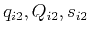, andare similar to those in the TI case for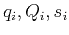, andfor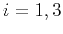. As a result, we need to specify parameter expressions only for the other two planes. Fitting both phase- and group-velocity expressions along both axes in each symmetry plane leads to six different expressions for each of the fitting parameters. This is different for the case of extended Muir-Dellinger approximations (equations 41 and 43) where only three expressions are allowed.

Since the Christoffel equation in the three symmetry planes is similar to that in TI media, the functional forms of every parameter expression remain the same. Therefore, we can compute parameters needed by equations 45 and 47 using the formulas derived in [,] plane for the TI case. Thus, we obtain:(51)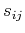(52)(53)where. Recall that the indicesand therefore, there are six expressions corresponding to each formula from equations 51-53. Equations 45 and 47 amount to the nine-parameter approximations for both velocities where the required parameters include,,,,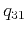,,,, andfor equation 45 or their reciprocals,,,,,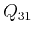,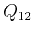,,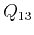, andfor equation 47. However, these nine parameters can be easily reduced to six using the linear relationships betweenandfor different lithologies from the previous discussion on TI media (Figure 1). Similar conversion rules apply for anisotropic parameters in each symmetry plane and are summarized in Table 1. Note that the required independent parameters for the group-velocity approximations derived based on Muir-Dellinger approach can be found in a one-to-one relationship simply from the reciprocals of the required parameters for phase-velocity approximations as mentioned before. Therefore, phase- and group-velocity approximations derived on the basis of this approach require exactly the same number of parameters.

SubsectionsOn anelliptic approximations for qP velocities in TI and orthorhombic mediaNext: Moveout approximation Up: Orthorhombic media Previous: Extended Muir-Dellinger Approximations

2017-04-14